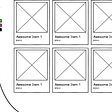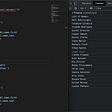# Big O Notation: part 2

This is the second part of a two-part article on big O notation using javascript, in case you missed it, the first part is here. The last article covered definitions and the time complexity of some sample functions. This one covers the complexity of some tricky functions, space complexity, and logarithms, let's get into it.

Let’s start by discussing the time complexity of a function

As the name suggests, this function takes a number as an argument and logs all integers from 1 to the number if it is less than five, if it is more than five, it logs 1 to 5. Considering the time complexity of this function, we have to look at what happens for arguments greater than five, integers are infinite but no matter the value of the integer passed, it does not print more than five values so looking at the big picture, it doesn't really matter that for values from 1 to 5 it is linear time because, for values greater than 5 which are infinite, it is constant time so the time complexity is O(1)

Another interesting function

This is kinda the opposite of the previous one as it logs at least five values from 1 so for every value below 5, it logs 5 values which is constant time but for values more than five, it logs from 1 to that number. As we established, we consider how the function behaves as the argument grows towards infinity so it does not matter that it is constant time for numbers less than 5 because numbers greater than 5 are infinite. The time complexity of this function is O(n). Note: for the two last functions, we only considered positive integers.

# Space Complexity

Space complexity is quite similar to time complexity. The notations do not look any different from the notations for time complexity, the only difference is that we are talking about space, not time. Note that in the context of this article, we are not considering the space that the arguments take up, we are only considering the space that the function itself takes to run. First, we have to understand that some data types in javascript take constant space, for example, numbers, so storing 3 in a variable takes up roughly the same space as storing 5 or 10. The same goes for booleans, undefined and null. Strings require O(n) space where n is the length of the string (not the number of characters as the length of some characters might be more than one for example emojis and some non-English characters). Arrays and Objects are O(n), where n is the length of the array for arrays or the number of keys for Objects. Let’s take an example.

This function takes an integer and returns the sum of integers from 1 to that number. There are two variables taking up space in this function, `sum` and `i` ,`i` is assigned a value once and `sum` is assigned a value n times where n is the value passed to the function, the value assigned to both variables are numbers meaning it takes up about the same space for any number so even though `sum` is reassigned a few times depending on the argument, it still takes up the same space so the space this function takes up is constant, making its space complexity O(1). Another function

This one takes an array of integers, adds 2 to every value in the array, and returns a new array containing the results. Remember the space taken to store arrays are proportional to the length so in this case, the more values the argument array has, the more values the resultant array would have, making the space complexity of this function linear written as O(n).

# Logarithms

You might remember logarithms from high school, if you don’t, don’t worry, I'll try to explain a bit. According to the English dictionary which also happens to be mathematically accurate,

a logarithm is a quantity representing the power to which a fixed number (the base) must be raised to produce a given number.

A logarithm is the inverse of exponentiation same way subtraction is the inverse of addition. If xʸ equals z then logₓ(z) (pronounced as log z base x) equals y. For example, 2⁴ = 16 so log₂(16) = 4, 2 must be raised by 4 to produce 16. When calculating complexities and in computer science generally, we tend to use logarithm in base 2 which is also called binary logarithm so you might just see something like log(x), in evaluating functions, it's most likely in base 2. By definition and at the most basic level, the binary logarithm of a number is the number of times you can divide that number by two before you get 1. So if we have 16, we divide by two, we get 8, again and we get 4 and then 2 and 1 so the binary logarithm is 4 cause we had to do 4 divisions by two to get 1. The binary logarithm of a number less than the number itself (for example, log 4 which equals 2 is less than 4). This means, by comparison, a function with a complexity of O(log n) is way better in performance than one with O(n).

An example of a function that would have a logarithmic time complexity is an implementation of the classic binary search algorithm. Let’s do some explanation and then we’ll see some code. The binary search algorithm is a search algorithm if that was not obvious. It works with a sorted dataset so let’s say we have an array of numbers that have been sorted in ascending order and we want to search for a value in the array. Rather than comparing one for one from the beginning to the end of the array until we find it or not, binary sort involves taking the value we are searching for and comparing it with the value at the center of the array, if it is greater, we disregard the values from the beginning to the center of the array, if it is less, we disregard the values from the center to the end of the array and if they are equal then voila, there’s our result. This works because the array is sorted so we can conclude that if the value we are searching for is greater than the one at the center, it is definitely greater than the values before the center, and if it is less than the one at the center, it is definitely less than the ones after the center. We keep comparing the center value and removing parts of the array until we are down to one value. If a particular array does not have a perfect center, we use the nearest index. This means that for every iteration of our search, we split the array into two so if we started with an array of 16 values, we’d be down to 8 and then 4 and 2 and 1, that’s four iterations. If we check the relationship between the length of the array (16), and the iterations we performed (4), we would notice that the number of iterations is the binary logarithm of the length of the array meaning a binary search algorithm has a time complexity of O(log n). Also, it’s possible that we do not have to complete these iterations before we get the value but we usually go for the worst-case scenario. Here’s an implementation of the binary search algorithm

Space complexity as you must’ve noticed is quite similar to time complexity, the same rules apply, we also have functions with quadratic space complexities and logarithmic ones just like with time. The calculations are all the same.

You can follow me for more articles on software engineering. I hope these articles were helpful, have fun evaluating functions 🙂.

--

--

--

## More from Prince Anyaoha

Engineer | MCU fan

Love podcasts or audiobooks? Learn on the go with our new app.

## How to do 3-legged OAuth with GitHub, a general guide by example with Node.js## Battle of the Colors## Custom Event with RxJS## Creating your first Node.js REST API with Nest and Typescript## How Microsoft Wants To Change TypeScript## I love elm and hate static typing languages## Prince Anyaoha

Engineer | MCU fan

## Simple Cipher Solution## Javascript, write it clean PART 1 : variables.## Breakdown: Fetch() Method